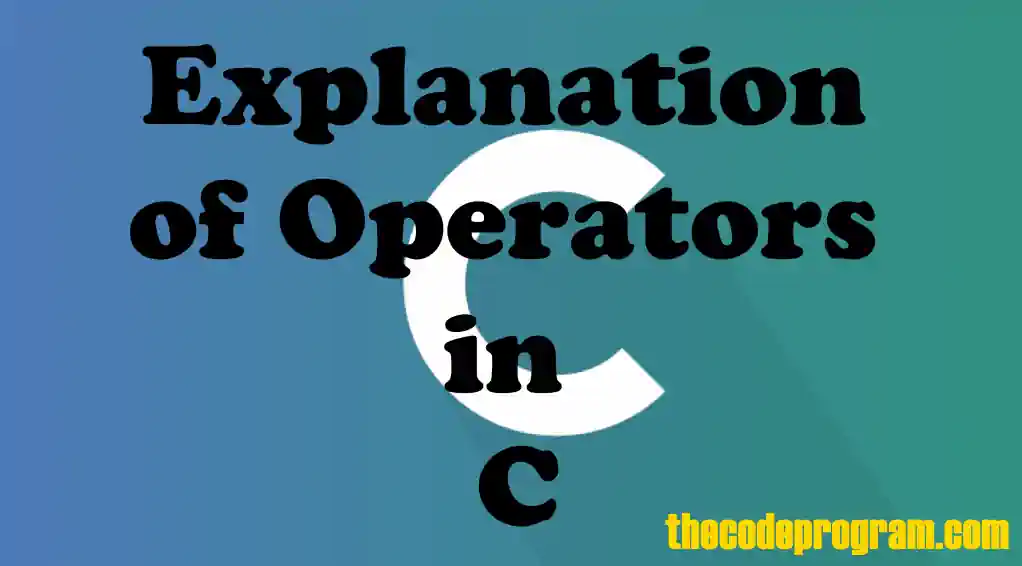## Explanation of Operators in C

Hello everyone, in this article we are going to talk about usages of operators in C programming language. We are going to talk what they are and then we make some examples on operators.

Let's begin

Firstly I need to say, what operators are ?
An operator instruct the compiler and so compoter to make some calculations. These calculations may be a logical or arithmetic calculations or other type mathematical operations. With these calculations we can manipulate the values to get another result.
There are 7 types operators exist. These are:
• Arithmetic operators This type of operators provide the arithmetical operations.
• Relational operators This type of operators provide the checking some relations between variables like equalizations, bigger or smaller status.
• Logical operators This operators provide the controlling the logical values.
• Bitwise operators This operators make some operations on the numbers at bit levels.
• Assignment operators This operators provides the assignmetns the right side value to the left side variable.
• Conditional operators This operator as we know check the condition of the variable. if/else-if is the most commonly known operator.

Let's start to talk one by one:

#### Arithmetic operators

We can perform arithmetical operations like adding, multipling, dividing or substracting with arithmetic operators. Below list you can see the arithmetic operators.

+ Performs adding two or more operands Performs subtracting right operand from the left operand multiplies two or more operands divide left operator by right operator return remain from dividing Increment : adds "1" to variable (+x : increase by x) Decrement - subtracts "1" from variable (+x : increase by x)
##### Examples
``````
int x = 5 + 7;      //returns 12
int y = 15 - 6;     //returns 9
int z = y++;        //returns 10
int t = x % y       //returns 2
``````

#### Relational operators

We can perform a checking the variables' relations like equality or NOT equality according to each other. Below list you can see the list of relational operators.

== Check the operands are equals Check the operands are not equals. Check the left operand greater then the right operand Check the right operand smaller than the right operand Check the right operand greater or equal to the right operand Check the left operand smaller or equal to the right operand
##### Examples
``````
a = 5;
b= 7;
if( a < b ) printf("a is smaller than b");
if( b > a ) printf("b is greater than a");

if( a == b) printf("a and b are equal");
else printf("a and b are not equal"); // this returns a and b are not equal
``````

#### Logical operators

We can perform logical operations with logical operators. We can use the only boolean terms. Only TRUE-FALSE or 1-0. Below list you can see the list of operators:

&& Performs AND operation between logic operands Performs OR operation between logic operands Performs NOT operation to a logic operand
##### Examples :
``````
boolean a = true;
boolean b= false;
boolean c = true;

a && b     // returns FALSE
a || b      // returns TRUE
!c              // returns FALSE
``````

#### Bitwise operators

We can perform the operations at the bit level of the numerical value with Bitwise operators. The operands first be converted to binary level and then make bitwise operations. We can not use this operators with double and float typed numbers. We can perform a multipling or dividing by 2 with a bitshifting or we can make a logical operation of all bits of two operand. Below list you can see the list of bitwise operators.

& Performs AND operations on an operand at bit level Performs OR operations on an operand at bit level Performs XOR operations on an operand at bit level Shifts the bits of left operands to left as much as right operand Shifts the bits of left operands to right as much as right operand
##### Examples:
``````
1111 & 1010    //Returns 1010
1000 | 1001    //Returns 1001
1100100 >> 2  //Returns  0011001
1001 ^ 1100    //Returns 1010
``````

#### Assignment operators

We can perform assigment operations with assignment opeators. We can perform the equalization. We can set the right side value of the operator to the left side variable. Below list you can see the list of operators.

= Assign right operand to the left operand Perform adding the right operand to left operand Perform a substraction from left operand to right operand Perform a multiplyng operation with left and right operand and assign the result to left operand Divide left side operand to the right side operand and assign the reslt to left operand back. Perform am mod() operation with left operand and right operand and assign result to left opeand back.
##### Examples :
``````
//Assignmetns the values to left operands
a = 5;
b = 6;
c = 7;

a++;    //Now variable  a is 6
b+=5;    //Now variable b is 11
c*=3     //Now variable c is 21
``````

#### Also C programming language has some important operators. Below you can see them

sizeof() This function operator returns the size of variable This sign is same with bitwise AND but this will comes at before of variable and it show the varable address This is a variable pointer This operators perform a conditional oparational. (variable = is condition true ? then X : else Y)

Have a good operations in C languages.

I wish you all healthy days.

Burak Hamdi TUFAN

16/04/2020

18/04/2020

27/02/2021